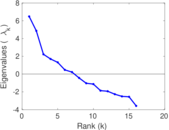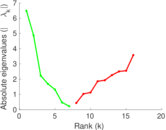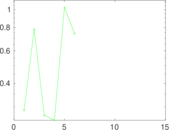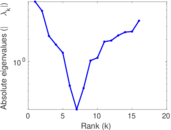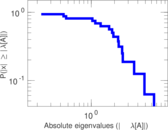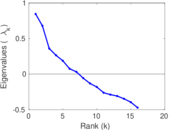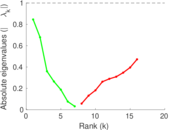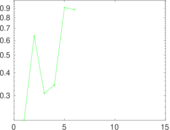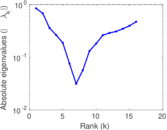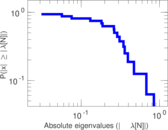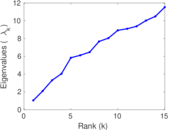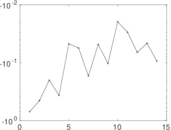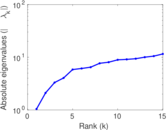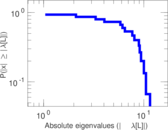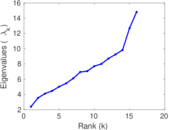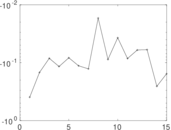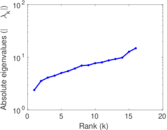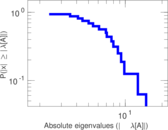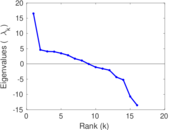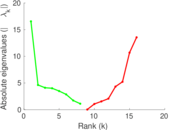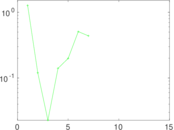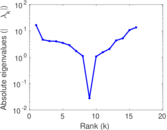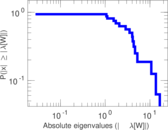# Highland tribes

This is the signed social network of tribes of the Gahuku–Gama alliance structure of the Eastern Central Highlands of New Guinea, from Kenneth Read (1954). The network contains sixteen tribes connected by friendship ("rova") and enmity ("hina").

 Code `HT` Internal name `ucidata-gama` Name Highland tribes Data source http://vlado.fmf.uni-lj.si/pub/networks/data/ucinet/ucidata.htm#gama AvailabilityDataset is available for download Consistency checkDataset passed all tests Category Human social network Node meaning Tribe Edge meaning Friendship Network format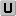Unipartite, undirected Edge type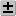Signed, possibly weighted, no multiple edges LoopsDoes not contain loops

## Statistics

 Size n = 16 Volume m = 58 Loop count l = 0 Wedge count s = 387 Claw count z = 777 Cross count x = 1,033 Triangle count t = 68 Square count q = 283 4-Tour count T4 = 3,928 Maximum degree dmax = 10 Average degree d = 7.250 00 Fill p = 0.483 333 Size of LCC N = 16 Diameter δ = 3 50-Percentile effective diameter δ0.5 = 0.979 675 90-Percentile effective diameter δ0.9 = 1.833 59 Median distance δM = 1 Mean distance δm = 1.454 21 Gini coefficient G = 0.131 466 Balanced inequality ratio P = 0.405 172 Relative edge distribution entropy Her = 0.988 424 Power law exponent γ = 2.182 81 Tail power law exponent γt = 8.991 00 Tail power law exponent with p γ3 = 8.991 00 p-value p = 0.410 000 Degree assortativity ρ = +0.049 904 8 Degree assortativity p-value pρ = 0.594 725 Clustering coefficient c = 0.527 132 Spectral norm α = 6.483 38 Algebraic connectivity a = 2.102 54 Spectral separation |λ1[A] / λ2[A]| = 1.332 48 Non-bipartivity bA = 0.561 989 Normalized non-bipartivity bN = 0.517 455 Algebraic non-bipartivity χ = 2.375 14 Spectral bipartite frustration bK = 0.081 901 4 Negativity ζ = 0.500 000 Algebraic conflict ξ = 1.040 29 Triadic conflict τ = 0.132 353 Spectral signed frustration φ = 0.035 872 0 Controllability C = 1 Relative controllability Cr = 0.062 500 0

## Plots

### Fruchterman–Reingold graph drawing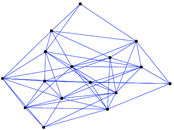### Degree distribution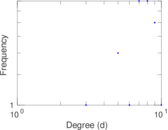### Cumulative degree distribution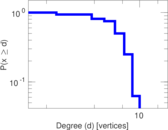### Lorenz curve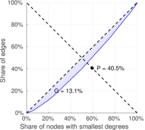### Spectral distribution of the adjacency matrix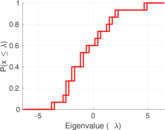### Spectral distribution of the normalized adjacency matrix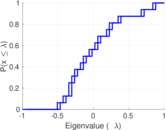### Spectral distribution of the Laplacian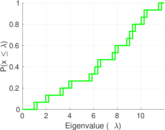### Spectral graph drawing based on the adjacency matrix### Spectral graph drawing based on the Laplacian### Spectral graph drawing based on the normalized adjacency matrix### Degree assortativity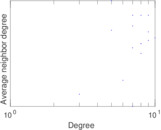### Zipf plot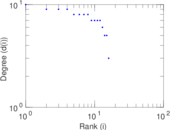### Hop distribution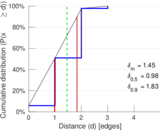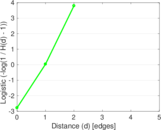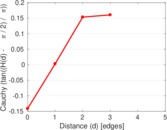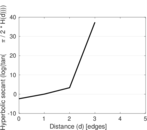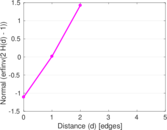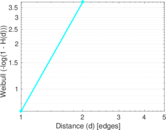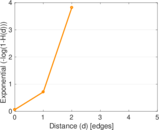### Double Laplacian graph drawing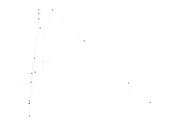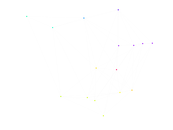### Delaunay graph drawing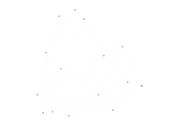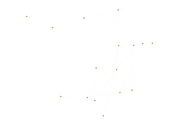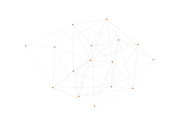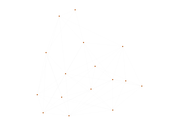### Item rating evolution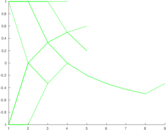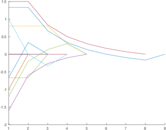### Edge weight/multiplicity distribution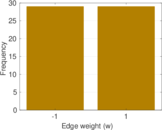### Clustering coefficient distribution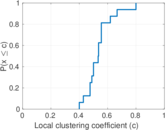### Average neighbor degree distribution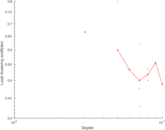### SynGraphy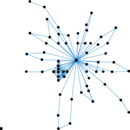### Matrix decompositions plots